## Example Questions

← Previous 1 3 4 5 6

### Example Question #781 : Act Science

The Ideal Gas Law is as follows:is pressure as measured in Pascals,is volume as measured in cubic meters,is the number of moles of the gas,is the gas constant known as 8.314 Joules per mole times Kelvin, and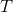is the temperature measured in Kelvin.

A class of students began studying the Ideal Gas Law and how the Pressure and the Volume relate to one another.  They took 20 moles of a sample gas and kept the room at a temperature of 300 Kelvin.  They then used different sized containers of the gas to limit and expand the volume.  At each different volume, they measure the pressure of the gas on its container.  The table they made from their results is seen in table 1.

 Volume of the container Pressure Measured in Pascals 1 cubic meter 49,884 Pascals 2 cubic meters 24,942 Pascals 3 cubic meters 16,628 Pascals 4 cubic meters 12,471 Pascals 5 cubic meters 9,976.8 Pascals 6 cubic meters 8,314 Pascals 7 cubic meters 7,126.2 Pascals

TABLE 1

And they graph their findings in Firgure 1.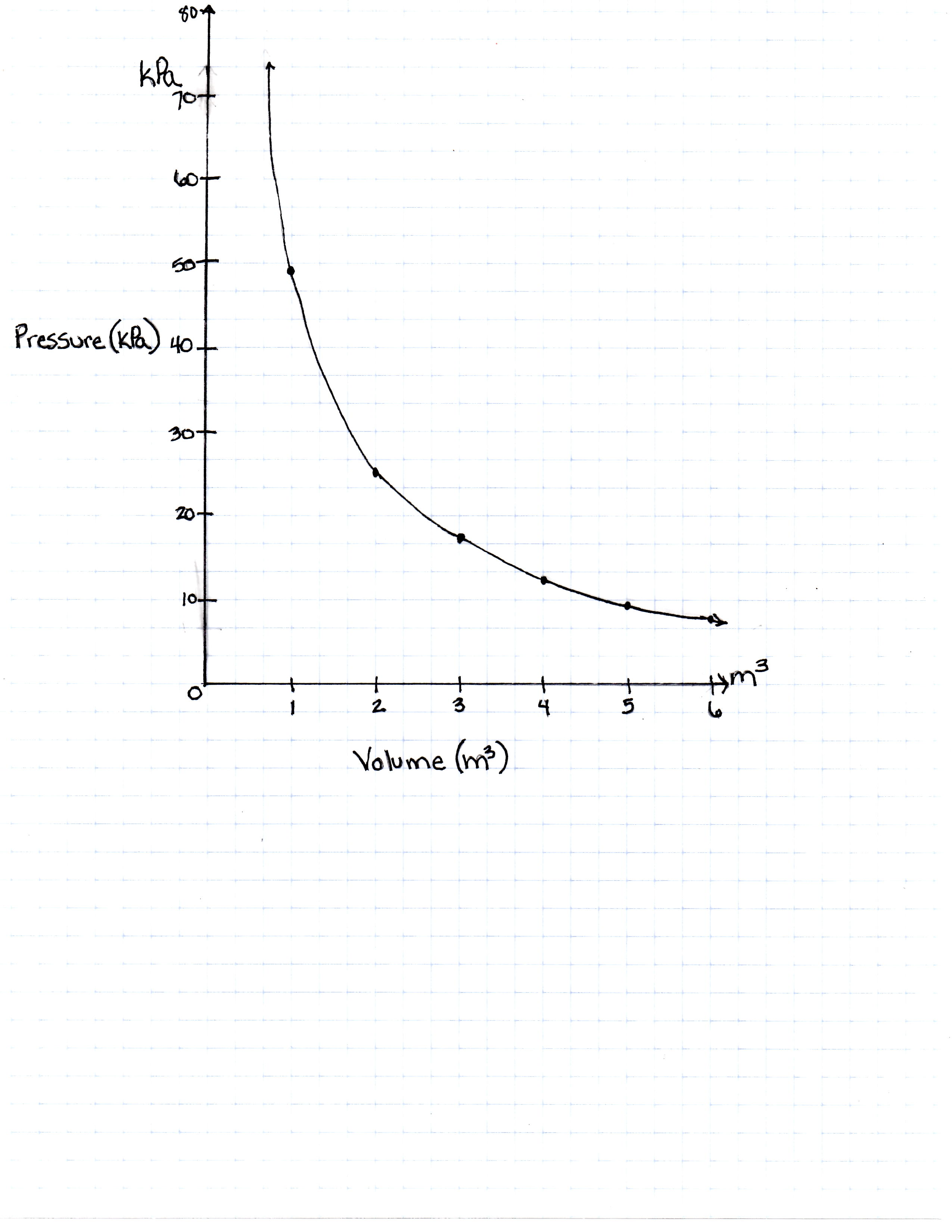FIGURE 1

If the same 20 moles of the same gas were taken to sea level (at a pressure of 100,000 Pascals) and heated to 300 Kelvin, how large would the volume of the container be to hold it at its new pressure?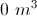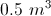Not enough information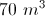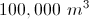Explanation:

Pressure increases as volume decreases. For the gas to be put at the air pressure at sea level, which is approximtely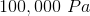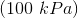, the volume must decrease.  The equation leads us to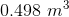, or approximately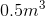.

### Example Question #1 : How To Find Synthesis Of Data In Chemistry

The rate of a reversible chemical reaction depends on many factors, including concentrations of the reactants and products, temperature, and presence of enzymes called catalysts. In the forward reaction, two reactants combine to form one product. However, in a reverse reaction, the product is broken down into the two reactants.

In order for a forward reaction to occur, the reactants moving around in the test tube must physically interact with each other. The more often reactants interact with each other, the more produce is formed in the same amount of time. The speed at which reactants combine into products (the rate of the reaction) can be calculated by dividing the amount of a chemical produced in a reaction (often measured in moles) by the time it takes to produce that amount.

In order to determine the effects of reactant and product concentration, temperature, and presence of catalysts on the rate of a reaction, a scientist studied the following reaction: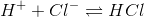The scientist varied the conditions of the experiment and measured the rate of the reaction. The results are outlined in Table 1. The units of concentration are moles per liter.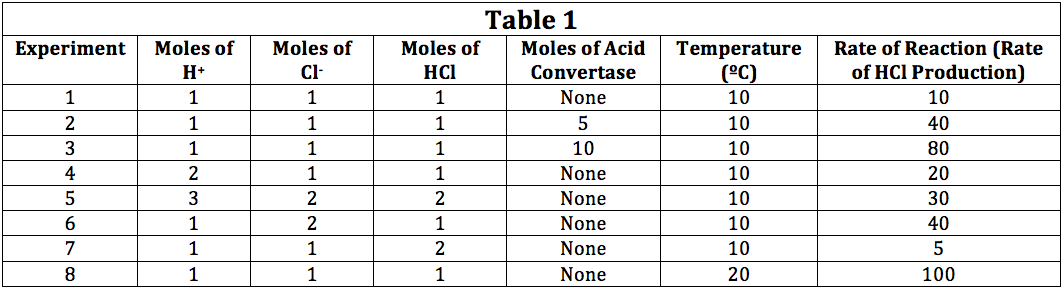In order to display the relationship between the number of moles of H+ and the rate of the reaction in Experiments 1 and 4, the scientist could:

Plot the number of moles of H+ against the moles of Cl-

Plot the number of moles of H+ against the moles of acid convertase

Plot the number of moles of H+ against the temperature

Plot the number of moles of H+ against the rate of the formation of HCl

Plot the number of moles of H+ against the rate of the formation of HCl

Explanation:

The question asks us to determine the best way to show the relationship between the moles of a certain reactant, H+, and the rate of the reaction. The most logical way would be to plot the moles versus the rate, providing a line or bar graph that would show how the rate increased with increasing concentration of the reactant. The other plot set ups do not provide a clear way to show how increasing or decreasing the concentration of a reactant would change the rate.

### Example Question #1 : How To Find Synthesis Of Data In Chemistry

The rate of a reversible chemical reaction depends on many factors, including concentrations of the reactants and products, temperature, and presence of enzymes called catalysts. In the forward reaction, two reactants combine to form one product. However, in a reverse reaction, the product is broken down into the two reactants.

In order for a forward reaction to occur, the reactants moving around in the test tube must physically interact with each other. The more often reactants interact with each other, the more produce is formed in the same amount of time. The speed at which reactants combine into products (the rate of the reaction) can be calculated by dividing the amount of a chemical produced in a reaction (often measured in moles) by the time it takes to produce that amount.

In order to determine the effects of reactant and product concentration, temperature, and presence of catalysts on the rate of a reaction, a scientist studied the following reaction:The scientist varied the conditions of the experiment and measured the rate of the reaction. The results are outlined in Table 1. The units of concentration are moles per liter.The effect of temperature on the rate of reaction is best demonstrated by comparing which two trials?

Trials 1 and 4

Trials 1 and 8

Trials 3 and 6

Trials 5 and 8

Trials 1 and 8

Explanation:

In Table 1, Trials 1 and 8 have the same number of moles of H+, Cl-, and HCl. Additionally, neither trial contains acid convertase. However, in Trial 8, we can see that the temperature was doubled from that of Trial 1. Thus, Trials 1 and 8 show the relationahip between temperature and reaction rate most clearly.

### Example Question #101 : Chemistry

The rate of a reversible chemical reaction depends on many factors, including concentrations of the reactants and products, temperature, and presence of enzymes called catalysts. In the forward reaction, two reactants combine to form one product. However, in a reverse reaction, the product is broken down into the two reactants.

In order for a forward reaction to occur, the reactants moving around in the test tube must physically interact with each other. The more often reactants interact with each other, the more produce is formed in the same amount of time. The speed at which reactants combine into products (the rate of the reaction) can be calculated by dividing the amount of a chemical produced in a reaction (often measured in moles) by the time it takes to produce that amount.

In order to determine the effects of reactant and product concentration, temperature, and presence of catalysts on the rate of a reaction, a scientist studied the following reaction:The scientist varied the conditions of the experiment and measured the rate of the reaction. The results are outlined in Table 1. The units of concentration are moles per liter.If a scientist desired to produce the most HCl possible in a reaction, the scientist could:

Increase the moles of both H+ and Cl-

Increase the moles of HCl

Remove acid convertase

Decrease the temperature

Increase the moles of both H+ and Cl-

Explanation:

Using Table 1 as a guide, we can see that the rate of the reaction increases when H+ and Cl- are individually doubled over Trial 1. Thus, it is reasonable to conclude that simultaneously increasing the moles of each of these would further increase the rate. The remaining options all have effects opposite that of maximizing the rate of reaction.

### Example Question #2 : How To Find Synthesis Of Data In Chemistry

The rate of a reversible chemical reaction depends on many factors, including concentrations of the reactants and products, temperature, and presence of enzymes called catalysts. In the forward reaction, two reactants combine to form one product. However, in a reverse reaction, the product is broken down into the two reactants.

In order for a forward reaction to occur, the reactants moving around in the test tube must physically interact with each other. The more often reactants interact with each other, the more produce is formed in the same amount of time. The speed at which reactants combine into products (the rate of the reaction) can be calculated by dividing the amount of a chemical produced in a reaction (often measured in moles) by the time it takes to produce that amount.

In order to determine the effects of reactant and product concentration, temperature, and presence of catalysts on the rate of a reaction, a scientist studied the following reaction:The scientist varied the conditions of the experiment and measured the rate of the reaction. The results are outlined in Table 1. The units of concentration are moles per liter.In Experiment 8, if the moles of H+ were doubled, the expected rate of the reaction would be:

> 250

< 80

<20

> 100

> 100

Explanation:

This question asks us to consider what the effect of doubling the moles of H+ would be on the rate of the reaction. However, we must take into consideration that Experiment 8 has a doubled temperature, making the base rate 100. If we consider Experiments 1 and 4, we can see that doubling the H+ concentration doubles the rate. Thus, we could predict that the rate would be somewhere around 200. The only option that fits the predicted value is a rate > 100.

### Example Question #3 : How To Find Synthesis Of Data In Chemistry

The rate of a reversible chemical reaction depends on many factors, including concentrations of the reactants and products, temperature, and presence of enzymes called catalysts. In the forward reaction, two reactants combine to form one product. However, in a reverse reaction, the product is broken down into the two reactants.

In order for a forward reaction to occur, the reactants moving around in the test tube must physically interact with each other. The more often reactants interact with each other, the more produce is formed in the same amount of time. The speed at which reactants combine into products (the rate of the reaction) can be calculated by dividing the amount of a chemical produced in a reaction (often measured in moles) by the time it takes to produce that amount.

In order to determine the effects of reactant and product concentration, temperature, and presence of catalysts on the rate of a reaction, a scientist studied the following reaction:The scientist varied the conditions of the experiment and measured the rate of the reaction. The results are outlined in Table 1. The units of concentration are moles per liter.The direction of the reaction most likely depends on:

The moles of Cl-

The moles of H+

The moles of HCl

Not enough information to determine

Not enough information to determine

Explanation:

The passage and Table 1 only describe the rate of formation of HCl, not whether the reaction primarily forms HCl or whether HCl is broken down into H+ and Cl-. Unless the passage described in more detail how HCl was formed, we cannot assume that the direction of the reaction is solely dependent on one of the factors listed in the table. While we can see that the rate of the reaction slows down when more HCl is initially present at the start of the reaction, we do not know if the reaction rate becomes negative at some point (meaning that more HCl is being broken down than formed).

### Example Question #781 : Act Science

Both gases and liquids are considered to be fluids that have individual molecules that move around with kinetic and potential energy. Kinetic energy, defined as the energy related to motion, takes three forms: translational energy that occurs as a molecule moves from position A to position B, rotational energy that occurs as a molecule spins around an imaginary axis at its center of mass, and vibrational energy that occurs as individual atoms in a molecular bond move towards and away from each other. Usually, molecules possess varying combinations of kinetic energy forms. In contrast, potential energy is defined as stored energy that could be released to become kinetic energy. The total energy of a molecule is fixed, meaning that a molecule has some combination of kinetic and potential energies.

Varying amount of kinetic and potential energies define how molecules in a fluid interact with each other. For example, when the kinetic energy of a molecule is high (greater than 1000J), it can no longer interact with neighboring molecules strongly enough to remain a liquid. However, if the potential energies are too high (greater than 1000 J), molecules cannot escape a liquid to become a gas. If the kinetic energy is high and the potential energy is low, molecules tend to become a gas and can be modeled by an equation known as the Ideal Gas Law: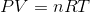Where P is the pressure of a gas, V is the volume, n is the number of moles of a gas, R is a constant, and T is temperature in degrees Kelvin.

The Ideal Gas Law perfectly applies to particles with no mass, no intermolecular interactions, and no true volume. However, real molecules do not adhere perfectly to the Ideal Gas Law.

If the kinetic and potential energies of a liter of oxygen molecule are 1500 J and 100 J, respectfully, the liter is most likely to be in what phase according to the passage?

Solid

Cannot Be Determined

Gas

Liquid

Gas

Explanation:

According to the second paragraph of the passage, we learn that molecules that have high kinetic energy (defined as > 1000 J) and low potential energy (< 1000 J) are less likely to interact with each other to form the liquid or solid phase. Thus, this leaves the gas phase for these molecules to inhabit.

### Example Question #782 : Act Science

Both gases and liquids are considered to be fluids that have individual molecules that move around with kinetic and potential energy. Kinetic energy, defined as the energy related to motion, takes three forms: translational energy that occurs as a molecule moves from position A to position B, rotational energy that occurs as a molecule spins around an imaginary axis at its center of mass, and vibrational energy that occurs as individual atoms in a molecular bond move towards and away from each other. Usually, molecules possess varying combinations of kinetic energy forms. In contrast, potential energy is defined as stored energy that could be released to become kinetic energy. The total energy of a molecule is fixed, meaning that a molecule has some combination of kinetic and potential energies.

Varying amount of kinetic and potential energies define how molecules in a fluid interact with each other. For example, when the kinetic energy of a molecule is high (greater than 1000J), it can no longer interact with neighboring molecules strongly enough to remain a liquid. However, if the potential energies are too high (greater than 1000 J), molecules cannot escape a liquid to become a gas. If the kinetic energy is high and the potential energy is low, molecules tend to become a gas and can be modeled by an equation known as the Ideal Gas Law:Where P is the pressure of a gas, V is the volume, n is the number of moles of a gas, R is a constant, and T is temperature in degrees Kelvin.

The Ideal Gas Law perfectly applies to particles with no mass, no intermolecular interactions, and no true volume. However, real molecules do not adhere perfectly to the Ideal Gas Law.

If the kinetic and potential energies of a liter of oxygen molecules are 250 J and 1200 J, respectfully, the liter is most likely to be in what phase according to the passage?

Cannot Be Determined

Solid

Gas

Liquid

Liquid

Explanation:

According to the second paragraph of the passage, molecules that have low kinetic energy and high potential energy interact with each other to form the liquid phase. The passage implies that they do not have enough energy to prevent interaction with one another and thus interact to form a liquid.

### Example Question #1 : How To Find Synthesis Of Data In Chemistry

Both gases and liquids are considered to be fluids that have individual molecules that move around with kinetic and potential energy. Kinetic energy, defined as the energy related to motion, takes three forms: translational energy that occurs as a molecule moves from position A to position B, rotational energy that occurs as a molecule spins around an imaginary axis at its center of mass, and vibrational energy that occurs as individual atoms in a molecular bond move towards and away from each other. Usually, molecules possess varying combinations of kinetic energy forms. In contrast, potential energy is defined as stored energy that could be released to become kinetic energy. The total energy of a molecule is fixed, meaning that a molecule has some combination of kinetic and potential energies.

Varying amount of kinetic and potential energies define how molecules in a fluid interact with each other. For example, when the kinetic energy of a molecule is high (greater than 1000J), it can no longer interact with neighboring molecules strongly enough to remain a liquid. However, if the potential energies are too high (greater than 1000 J), molecules cannot escape a liquid to become a gas. If the kinetic energy is high and the potential energy is low, molecules tend to become a gas and can be modeled by an equation known as the Ideal Gas Law:Where P is the pressure of a gas, V is the volume, n is the number of moles of a gas, R is a constant, and T is temperature in degrees Kelvin.

The Ideal Gas Law perfectly applies to particles with no mass, no intermolecular interactions, and no true volume. However, real molecules do not adhere perfectly to the Ideal Gas Law.

At a constant volume, the relationship between pressure and temperature as presented in the passage may best be depicted as: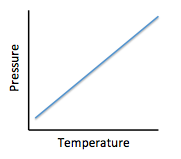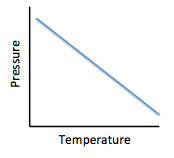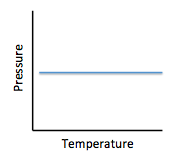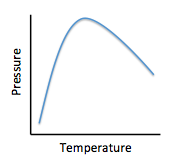Explanation:

Using the provided formula, we see that if volume is held constant, the relationship between pressure and temperature is a direct relationship. We know this because both are in the numerator on their respective sides of the equals sign. Thus, as pressure increases, we would also expect temperature to increase. A positive, straight line serves as the guide for this relationship.

### Example Question #2 : How To Find Synthesis Of Data In Chemistry

Both gases and liquids are considered to be fluids that have individual molecules that move around with kinetic and potential energy. Kinetic energy, defined as the energy related to motion, takes three forms: translational energy that occurs as a molecule moves from position A to position B, rotational energy that occurs as a molecule spins around an imaginary axis at its center of mass, and vibrational energy that occurs as individual atoms in a molecular bond move towards and away from each other. Usually, molecules possess varying combinations of kinetic energy forms. In contrast, potential energy is defined as stored energy that could be released to become kinetic energy. The total energy of a molecule is fixed, meaning that a molecule has some combination of kinetic and potential energies.

Varying amount of kinetic and potential energies define how molecules in a fluid interact with each other. For example, when the kinetic energy of a molecule is high (greater than 1000J), it can no longer interact with neighboring molecules strongly enough to remain a liquid. However, if the potential energies are too high (greater than 1000 J), molecules cannot escape a liquid to become a gas. If the kinetic energy is high and the potential energy is low, molecules tend to become a gas and can be modeled by an equation known as the Ideal Gas Law:Where P is the pressure of a gas, V is the volume, n is the number of moles of a gas, R is a constant, and T is temperature in degrees Kelvin.

The Ideal Gas Law perfectly applies to particles with no mass, no intermolecular interactions, and no true volume. However, real molecules do not adhere perfectly to the Ideal Gas Law.

At a constant temperature, the relationship between pressure and volume is best illustrated as: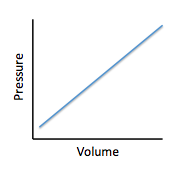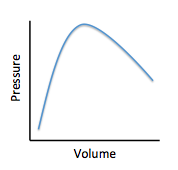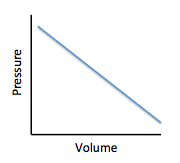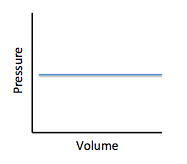Using the provided formula, we see that at a fixed temperature, pressure is inversely proportional to volume. They are on the same side of the equals sign, but can better be equated by rearranging the equation as: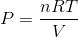. We can now see more clearly that pressure is inversly proportional to volume. This inverse relationship is depicted as a negative sloped linear curve.